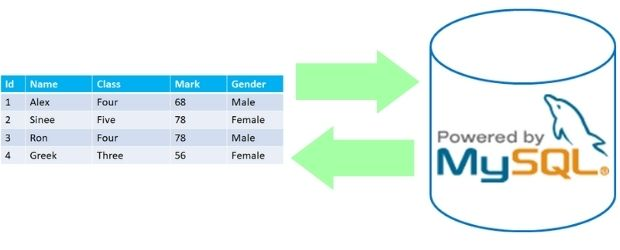# read_sql to get MySQL data to DataFrameBefore collecting data from MySQL , you should have Python to MySQL connection and use the SQL dump to create student table with sample data.

We will use `read_sql` to execute query and store the details in Pandas DataFrame.
 `sql` Query or name of the database table to collect data to DataFrame `con` Database connection string `params` default = None, Parameters to be passed along with the Query `index_col` Column to be used as index in DataFrame `coerce_float` bool, Converts non-string, non-numeric to float `parse_date` default=None, List of columns to be parse as Date `columns` List of columns to return, by default all columns are available. This option is to be used when in place of SQL table name is used. ( for reading only ) `chunksize` Number of rows to be included on each Chunk, iterator is returned.

read_sql to create Pandas DataFrame by using query from MySQL database table with options.

``````import mysql.connector
import pandas as pd
my_conn = mysql.connector.connect(
host="localhost",
user="userid",
passwd="******",
database="my_tutorial"
)
####### end of connection ####
my_data = pd.read_sql("SELECT * FROM student WHERE class='four'",my_conn)
print(my_data)``````
Output is here
``````   id         name class  mark     sex
0   1     John Deo  Four    75  female
1   4   Krish Star  Four    60  female
2   5    John Mike  Four    60  female
3   6    Alex John  Four    55    male
4  10     Big John  Four    55  female
5  15     Tade Row  Four    88    male
6  16        Gimmy  Four    88    male
7  21   Babby John  Four    69  female
8  31  Marry Toeey  Four    88    male``````
To get only specified columns
``print(my_data[['name','class']])``
( Query can be changed to retrieve only required columns )

## sqlalchemy

We can use SQLAlchemy database connection to get data to DataFrame.
``````import pandas as pd
from sqlalchemy import create_engine
my_data = pd.read_sql("SELECT * FROM student WHERE class='four'",my_conn)
print(my_data)``````

## Passing parameters to query

We will use params to pass parameters to our query
``````query="SELECT * FROM student WHERE class=%s"
Note that params takes list or tuple or dictionary. In the above code we used one tuple ( note the comma at the end of the tupple).
These are valid codes using different types of params
``````query="SELECT * FROM student WHERE class=%s"
Using List
``````query="SELECT * FROM student WHERE class=%s"
Using more parameters with SQL in ( read more on WHERE query )
``````query="SELECT * FROM student WHERE class=%s and id in(%s,%s)"
print(my_data)``````

## index_col

We can specify the index column to be used.
``````query="SELECT * FROM student WHERE class='Five'"
print(my_data)``````
Output ( DataFrame uses the id column as index column )
``````           name class  mark gender
id
7   My John Rob  Five    78   male
8        Asruid  Five    85   male
18        Honny  Five    75   male``````

## columns

Name of the columns to be returned. This is to be used when table name is used in place of any Query and in read mode only. Here student is our table name.
``````my_data=pd.read_sql('student',my_conn,columns=['id','name'])
print(my_data)``````
Output ( sample rows only, there are more records )
``````    id         name
0    1     John Deo
1    2     Max Ruin
2    3       Arnold
3    4   Krish Star
-----
-----``````
to get all columns
``````my_data = pd.read_sql("student",my_conn)
print(my_data.columns)``````
To print all column names by looping
``````my_data = pd.read_sql("student",my_conn)
print(my_data.columns) # output
for i in my_data.columns:
print(i)``````

## chunksize

We can get an iterator by using chunksize in terms of number of rows of records.
``````query="SELECT * FROM student "
print(next(my_data))
print("--End of first set of  records ---")
print(next(my_data))``````
Output is here
``````
id      name  class  mark     sex
0   1  John Deo   Four    75  female
1   2  Max Ruin  Three    85    male
2   3    Arnold  Three    55    male
--End of first set of  records ---
id        name class  mark     sex
0   4  Krish Star  Four    60  female
1   5   John Mike  Four    60  female
2   6   Alex John  Four    55    male``````

## Creating table with primary key , unique key

We can create table in our MySQL database and add primary key and unique key to columns. We can add other constraints to columns also.
``````query="CREATE TABLE IF NOT EXISTS student5 (`id` bigint(20) NOT NULL,\
`name` text,`class` text,`mark` bigint(20) DEFAULT NULL,gender text,\
PRIMARY KEY (`id`),UNIQUE KEY `id` (`id`)) ENGINE=InnoDB DEFAULT
CHARSET=latin1;"
``````
We can run multiple queries
``````query = query + query2
In above sample codes you can see the basic connection and execution remain same and only by changing query we can get data in different ways and types. Some of the sample queries are listed here .
``````#To get all records of a particular class
query="SELECT * FROM student WHERE class='Five'"

#To get highest mark among all records
query="SELECT max(mark) FROM student"

#To get average mark among all records
query="SELECT avg(mark) FROM student"

#To get highest mark of each class
query="SELECT class,count(*) as no FROM student GROUP BY class"

#To get average mark of each class
query="SELECT class,avg(*) as avg FROM student GROUP BY class"

#To get minimum mark of each class
query="SELECT class,min(*) as min FROM student GROUP BY class"

#To get record details of highest class
query="SELECT * FROM student WHERE class='Five' ORDER BY mark DESC LIMIT 0,1"

#To get second highest record of any class.
query="SELECT * FROM student WHERE class='Five' ORDER BY mark DESC LIMIT 1,1"``````
You can read more on various SQL in our SQL Exercise section.

Subscribe to our YouTube Channel here

## Subscribe

* indicates required
Subscribe to plus2netplus2net.com